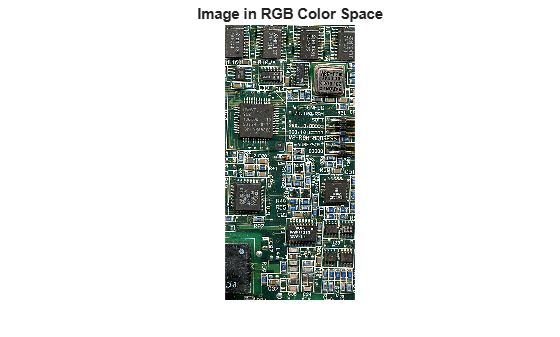# rgb2ycbcr

Convert RGB color values to YCbCr color space

## Syntax

``YCBCR = rgb2ycbcr(RGB)``

## Description

example

````YCBCR = rgb2ycbcr(RGB)` converts the red, green, and blue values of an RGB image to luminance (Y) and chrominance (Cb and Cr) values of a YCbCr image.```

## Examples

collapse all

Convert Image from RGB to YCbCr

Read an RGB image into the workspace.

`RGB = imread('board.tif');`

Convert the image to YCbCr.

`YCBCR = rgb2ycbcr(RGB);`

Display the original image and the new image

```figure imshow(RGB); title('Image in RGB Color Space');``````figure imshow(YCBCR); title('Image in YCbCr Color Space');```Convert Colormap from RGB to YCbCr.

Load an indexed image into the workspace. The colormap is in RGB colorspace.

`[I,map] = imread('forest.tif');`

Convert the colormap to YCbCr.

`newmap = rgb2ycbcr(map);`

Display the grayscale image with the original map and with the new map.

```figure imshow(I,map) title('Indexed Image with RGB Colormap');``````figure imshow(I,newmap) title('Indexed Image with YCbCr Colormap');```## Input Arguments

collapse all

RGB color values to convert, specified as a numeric array in one of these formats.

• c-by-3 colormap. Each row specifies one RGB color value.

• m-by-n-by-3 image

Data Types: `single` | `double` | `uint8` | `uint16`

## Output Arguments

collapse all

Converted YCbCr color values, returned as a numeric array of the same size as the input.

• If the input is `double` or `single`, then `Y` is in the range [16/255, 235/255] and `Cb` and `Cr` are in the range [16/255, 240/255].

• If the input is `uint8`, then `Y` is in the range [16, 235] and `Cb` and `Cr` are in the range [16, 240].

• If the input is `uint16`, then `Y` is in the range [4112, 60395] and `Cb` and `Cr` are in the range [4112, 61680].

 Poynton, C. A.A Technical Introduction to Digital Video, John Wiley & Sons, Inc., 1996, p. 175.

 Rec. ITU-R BT.601-5, Studio Encoding Parameters of Digital Television for Standard 4:3 and Wide-screen 16:9 Aspect Ratios, (1982-1986-1990-1992-1994-1995), Section 3.5.